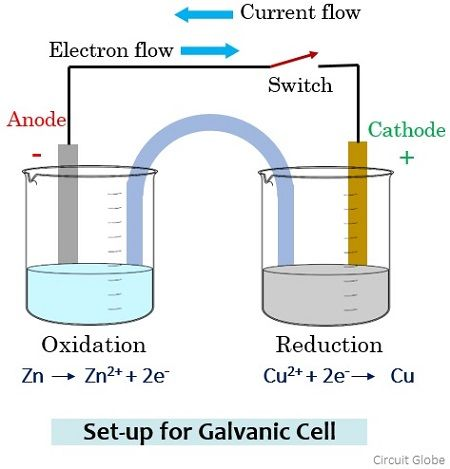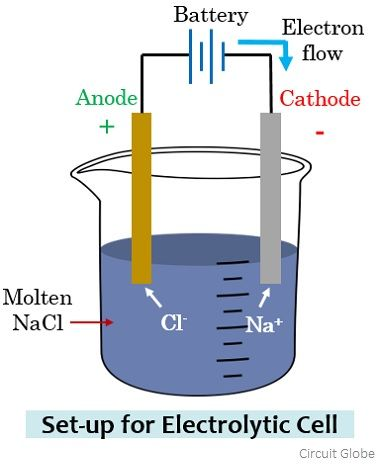# Difference Between Anode and Cathode

Anode and Cathode are the two classifications in which electrodes are classified. The significant difference between anode and cathode is that at anode oxidation takes place. As against, at the cathode, reduction occurs.

People generally in misconception regards anode particularly as positive and cathode particularly as negative. But in this content, you will get to know that the differentiation between anode and cathode is not merely done according to the type of polarity. But first, see-

What is an Electrode?

A crucial component of an electrochemical cell that makes contact with electrolyte is known as an electrode. Electrode acts as a metallic contact through which the current enters and leaves the electrolyte. More specifically, we can say, it is regarded as a surface where a redox reaction takes place between the metal and the solution.

Electrode generally represents an electrical conductor/ semiconductor within the electrochemical cell. It specifies the conductive phase where the transfer of charged carriers takes place.

An electrode that loses electrons and is accepted by the electrolyte undergoes oxidation. However, when the reverse operation occurs i.e. when the electrode gains electrons which are released by the electrolyte undergoes reduction.

## Content: Anode Vs Cathode

### Comparison chart

Basis for ComparisonAnodeCathode
BasicThe electrode where oxidation occurs.The electrode where reduction occurs.
Terminal polarity in electrolytic cellPositiveNegative
Terminal polarity in galvanic cellNegative Positive
BehaviourAn anode in electrolytic cell attracts anions.A cathode in electrolytic cell attracts cations.
NatureIn electrolytic cell it is a source of positive charge or electron acceptor.In electrolytic cell, it is a source of negative charge or electron donor.

### Definition of Anode

An anode is a type of electrode that can be of either positive polarity or negative polarity depending upon the type of cell. However, the anode is specifically defined as the electrode where oxidation i.e., loss of electrons takes place.

It is to be noted here that one can never define anode specifically as positive or negative in general as its polarity shows dependency on the type of cell.

### Definition of Cathode

Similar to an anode, a cathode can hold positive as well as negative charge depending upon cell type. For the cathode, it is said that it is an electrode where reduction i.e., the gain of electrons takes place.

Just like an anode, even a cathode can also be not defined according to its positive or negative polarity but the occurrence of reduction at an electrode implies that it is the cathode.

## Key Differences Between Anode and Cathode

1. The key factor of differentiation between anode and cathode is that anode corresponds to the electrode where oxidation i.e., loss of electrons occurs. While cathode corresponds to the electrode where reduction i.e., the gain of electrons occurs.
2. The specific denotation of the anode as positive and cathode as negative is wrong. This is so because the terminal polarity varies according to the type of cell i.e., electrolytic or galvanic in use.
3. For an electrolytic cell, anode acts as a positive terminal while a cathode holds negative polarity. Thus, an anode attracts negatively charged particles while a cathode attracts positively charged particles.
4. For a galvanic cell, anode holds the negative polarity whereas cathode acts as a positive terminal. Hence, here the anode will attract positively charged particles and the cathode will attract the negatively charged particles.

### Experimental Analysis

Consider a galvanic cell arrangement shown below in order to understand how the flow of current through the solution takes place.Here in the two separate beakers, we have copper sulphate and zinc sulphate solution. To maintain electric contact between the two solutions a salt bridge containing potassium chloride is used. The two electrodes of zinc and copper that will act as anode and cathode are connected by a metallic wire through a switch.

During the open condition of the switch, due to the open circuit arrangement, no reaction will occur in any of the beakers and so there will be no flow of current through the wire. Further, the switch is in the on state and we will get the closed-circuit arrangement then the electrons from the Zn electrode migrate (oxidation) through the salt bridge and get reduced at the Cu electrode (reduction).

The movement of anions (negatively charged particles) generates a current which flows through the metallic wire. However, the direction of the flow of current will be opposite to the flow of current.

As you have noticed here that among the two electrodes, oxidation occurs at the Zinc electrode thus is anode with negative polarity and reduction occurs at the Copper electrode thus is cathode with positive polarity in a galvanic cell arrangement.

However, on considering the electrolytic cell, the anode and cathode terminal polarity will get reversed. Let us understand this by considering an electrolytic cell arrangement shown below:Here sodium chloride in the molten state is taken into which a pair of electrodes are immersed. In the molten state, Na+, and Cl ions get separated and are in the free state. Along with this, the two electrodes are connected by a battery.

The electrode connected with the negative terminal of the battery attracts the Na+ ions while the anions i.e., Cl flows towards the electrode connected with the positive terminal. On reaching the respective electrode, the battery potential allows the gaining of electrons (reduction) by the Na+ ions, forming sodium metal.

Na+ + e = Na

Similarly, the Cl ions lose electrons (oxidation) at the electrode connected with the negative terminal resulting in Cl2 gas. Here the positive electrode where oxidation is occurring is the anode and the electrode where reduction is occurring is the cathode.

2 Cl = Cl2 + 2e

It is to be noted here that as electrons are moving from cathode to anode here thus the direction of the flow of current will be from anode towards the cathode.

The flow of current through molten sodium chloride leads to its decomposition into its elements i.e., sodium metal and chlorine gas.

### Conclusion

Recently we have seen that there are two types of electrochemical cells i.e., galvanic and electrolytic. The direction of flow of current is opposite to the direction in which the negatively charged particle moves. In an electrolytic cell, current flows from anode to cathode. While in a galvanic cell, the direction of the flow of current is from cathode to anode.

### 2 thoughts on “Difference Between Anode and Cathode”

1. Thank you so much. This helped me a lot in clearing my doubts , and now my concept is crystal clear. Thank you a million times.

2. Thank you, I appreciate all the effort you put to deliver us this kind of short notes. They are more easy to remember.